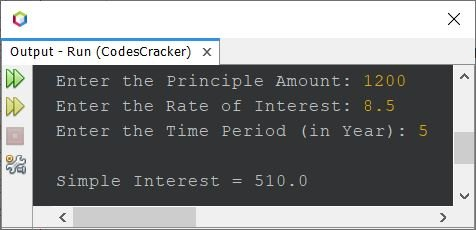# Java Program to Calculate Simple Interest

This article contains a program in Java to find and print simple interest based on the data entered by the user at runtime. But before creating the program, let's remind ourselves of the formula to calculate simple interest.

The formula to calculate simple interest is:

`SI = (P*R*T)/100`

where SI refers to the simple interest amount, P refers to the principle amount, R refers to the rate of interest, and T refers to the time period in years.

## Compute Simple Interest in Java

The question is, "Write a Java program to compute simple interest based on principle, rate, and time period entered by the user at run-time of the program." The program given below is an answer to this question:

```import java.util.Scanner;

public class CodesCracker
{
public static void main(String[] args)
{
float p, r, t, si;
Scanner scan = new Scanner(System.in);

System.out.print("Enter the Principle Amount: ");
p = scan.nextFloat();
System.out.print("Enter the Rate of Interest: ");
r = scan.nextFloat();
System.out.print("Enter the Time Period (in Year): ");
t = scan.nextFloat();

si = (p*r*t)/100;
System.out.println("\nSimple Interest = " +si);
}
}```

Here is its sample run with user input: 1200 as principle amount, 8.5 as rate of interest, and 5 as number of years or time period (in years):The program starts by importing the Scanner class from the java.util package, which is used to read user input.

The program defines a public class called CodesCracker with a public static method called main that takes an array of strings as input.

The main method initializes four variables of type float (p, r, t, and si) to store the principle amount, rate of interest, time period, and simple interest, respectively.

The program creates a new Scanner object called scan to read user input from the console.

The program prompts the user to enter the principle amount using the print() method of the System.out object and stores the user's input in the variable p using the nextFloat() method of the Scanner object.

The program prompts the user to enter the rate of interest and time period in a similar fashion and stores them in the variables r and t respectively.

The program calculates the simple interest by multiplying the principle amount, rate of interest, and time period, and then dividing the result by 100. The calculated value is stored in the variable si.

Finally, the program uses the println() method of the System.out object to print the calculated simple interest to the console, along with a message that indicates what the value represents.

Java Online Test

« Previous Program Next Program »